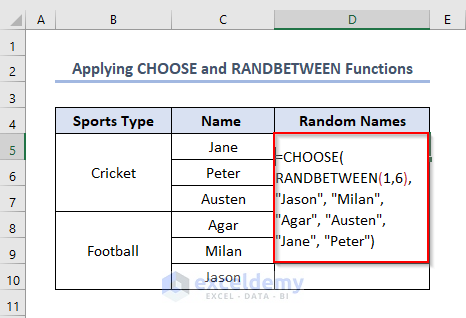# How to Select Names Randomly in Excel (5 Useful Methods)

Get FREE Advanced Excel Exercises with Solutions!

If you are looking for how to randomly select names in Excel, then you are in the right place. During the use of Excel we often need to select names randomly. Excel offers various functions by which we can select those names. In this article we’ll try to discuss how to randomly select names in Excel.

## How to Select Names Randomly in Excel: 5 Methods

Excel offers various methods to select names randomly. To show this, we have made a dataset named List of Players. The dataset is like this.### 1. Using RAND Function to Randomly Select Names in Excel

We can use the RAND function to select names randomly from a list without duplication.

• Firstly, write the following formula in the D5 cell like this.
`=RAND()`• Secondly, press ENTER and then use Fill Handle by dragging the cursor while holding it to the right bottom corner of the D5.• Eventually, we’ll get the outputs in the Random Column like this.
• This column is needed to select names randomly.• Thirdly, write the following formula using the INDEX and RANK functions in the E5 cell like this.
`=INDEX(\$C\$5:\$C\$14,RANK(D5,\$D5:\$D\$14))`

Formula Explanation

• RANK(D5,\$D5:\$D\$14)) → returns the rank of D5 e.0.640365959 among the values of cells D5:D14.
• \$C\$5:\$C\$14,RANK(D5,\$D5:\$D\$14)) →firstly returns the names in cells C5:C14 and then gives the rank of the name in D5 cell i.e. gives the rank of Jane.
• INDEX(\$C\$5:\$C\$14,RANK(D5,\$D5:\$D\$14)) → returns the intercept name which happens from the interception of the output value of \$C\$5:\$C\$14,RANK(D5,\$D5:\$D\$14)) and the rows from C5:C14.• Fourthly, press ENTER to get the output. Additionally, we can use Fill Handle to get other names randomly.### 2. Utilizing INDEX, RANDBETWEEN and COUNTA Functions to Randomly Select Names in Excel

We can use the combination of INDEX, RANDBETWEEN and COUNTAfunctions to select names randomly from a list. To show this, firstly, write the following formula in the D5 cell.

`=INDEX(\$C\$5:\$C\$14,RANDBETWEEN(1,COUNTA(\$C\$5:\$C\$14)),1)`

Formula Explanation

• COUNTA(\$C\$5:\$C\$14) → returns the number of counts from cell C5 to C14.
• Output10
• RANDBETWEEN(1,COUNTA(\$C\$5:\$C\$14)) → returns any random value between 1 and
• INDEX(\$C\$5:\$C\$14,RANDBETWEEN(1,COUNTA(\$C\$5:\$C\$14)),1) → returns the intercept name which happens from the interception of the output value of RANDBETWEEN(1,COUNTA(\$C\$5:\$C\$14)) and the rows from C5:C14.• Secondly, press ENTER to get the output Milan as a random name.• Thirdly, use Fill Handle to get multiple outputs of random selected names.### 3. Using INDEX, RANDBETWEEN and ROWS Functions

We can use INDEX, RANDBETWEEN and ROWS functions if we want to select a single random name. We need to write the formula in the D5 cell like this.

`=INDEX(\$C\$5:\$C\$14,RANDBETWEEN(1,ROWS(\$C\$5:\$C\$14)))`

Formula Explanation

• ROWS(\$C\$5:\$C\$14)→ Returns number of rows between cells C5 to C14.
• RANDBETWEEN(1,ROWS(\$C\$5:\$C\$14))→ Returns a random number between 1 and number of rows.
• INDEX(\$C\$5:\$C\$14,RANDBETWEEN(1,ROWS(\$C\$5:\$C\$14)))→ The number returned by RANDBETWEEN is fed to the row_num argument of the INDEX function, so it picks the value from that row. In the column_num argument, we supply 1 because we want to extract a value from the first column.• Press ENTER to get the random name as output.### 4. Applying CHOOSE and RANDBETWEEN Functions to Randomly Select Names in Excel

Excel allows us to get a random number from a range using CHOOSE and RANDBETWEEN functions. We need to write the formula in the D5 cell like this.

`=CHOOSE(RANDBETWEEN(1,6), "Jason", "Milan", "Agar", "Austen", "Jane", "Peter")`

The parameter bottom of the RANDBETWEEN function is 1 and the top is 6. The result of this function is a random number between 1 and 6 which is the index_num parameter of the CHOOSE function. The values of the function are “Jason”, “Miller”, “Agar”, “Austen”, “Jane”, “Peter”.

###• Secondly, press ENTER to find the output as a random name.Read More: Excel VBA: Random Selection from List

### 5. Using INDEX, RANK.EQ and COUNTIF Functions

We can also use INDEX, RANK.EQ and COUNTIF functions. RANK.EQ function is an improved version of the RANK function.
Firstly, fill up the Random Column by using the RAND function.
Secondly, write the following formula in the E5 cell.

`=INDEX(\$C\$5:\$C\$14,RANK.EQ(D5,\$D\$5:\$D\$14)+COUNTIF(\$D\$5:D5,D5)-1,1)`• Secondly, press ENTER and use Fill Handle to get all the outputs as random names.## Things to Remember

• If we use the combination of INDEX, RANDBETWEEN and COUNTA functions, we can get random names with duplicates i.e. one name can repeat several times.
• Using the RAND function gives the random names without duplication.

## Conclusion

We can select random names from a list of names if we study this article properly.

## What is ExcelDemy?

ExcelDemy Learn Excel & Excel Solutions Center provides free Excel tutorials, free support , online Excel training and Excel consultancy services for Excel professionals and businesses. Feel free to contact us with your Excel problems.Shajratul Alam Towhid

Hello, myself Shajratul Alam Towhid. Basically, I am a Naval Architect who wants to expand knowledge in the field of Microsoft Excel. I wish all of my articles will be beneficial for the readers.

We will be happy to hear your thoughtsAdvanced Excel Exercises with Solutions PDF